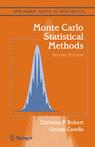## Le Monde puzzle [#1110]

Posted in Books, Kids, R, Travel with tags , , , , , , , , , on September 16, 2019 by xi'anA low-key sorting problem as Le Monde current mathematical puzzle:

If the numbers from 1 to 67 are randomly permuted and if the sorting algorithm consists in picking a number i with a position higher than its rank i and moving it at the correct i-th position, what is the maximal number of steps to sort this set of integers when the selected integer is chosen optimaly?

As the question does not clearly state what happens to the number j that stood in the i-th position, I made the assumption that the entire sequence from position i to position n is moved one position upwards (rather than having i and j exchanged). In which case my intuition was that moving the smallest moveable number was optimal, resulting in the following R code

```sorite<-function(permu){ n=length(permu) p=0 while(max(abs(permu-(1:n)))>0){
j=min(permu[permu<(1:n)])
p=p+1
permu=unique(c(permu[(1:n)<j],j,permu[j:n]))}
return(p)}
```

which takes at most n-1 steps to reorder the sequence. I however checked this insertion sort was indeed the case through a recursive function

```resorite<-function(permu){ n=length(permu);p=0 while(max(abs(permu-(1:n)))>0){
j=cand=permu[permu<(1:n)]
if (length(cand)==1){
p=p+1
permu=unique(c(permu[(1:n)<j],j,permu[j:n]))
}else{
sol=n^3
for (i in cand){
qermu=unique(c(permu[(1:n)<i],i,permu[i:n]))
rol=resorite(qermu)
if (rol<sol)sol=rol}
p=p+1+sol;break()}}
return(p)}
```

which did confirm my intuition.

## Le Monde puzzle [#1109]

Posted in Kids, R with tags , , , , , on September 4, 2019 by xi'anA digital problem as Le Monde current mathematical puzzle:

Noble numbers are such that they only involve different digits and are multiple of all their digits. What is the largest noble number?

Hmmmm…. Brute force? Since the maximal number of digits is 10, one may as well try:

```k=soz=9
for (t in 1:1e3){
sol=1
while (sol<10^(k-1)){
u=sample(0:8,k);i=digit2int(u)
if (max(i%%u[u>0])==0) soz=max(soz,sol<-i)}}
```

which returns 9875643120… (I made the conscious choice to exclude zero from the dividers. Which was not a choice spelled out in the original question.)

## Le Monde puzzle [#1107]

Posted in Kids with tags , , , on July 8, 2019 by xi'anA light birthday problem as Le Monde mathematical puzzle:

Each member of a group of 35 persons writes down the number of those who share the same birth-month and the number of those who share the same birth-date [with them]. It happens that these 70 numbers include all integers from 0 to 10. Show that at least two people share a birth-day. What is the maximal number of people for this property to hold?

Which needs no R code since the result follows from the remark that the number of individuals sharing a birth-month with just one other, n¹, is a multiple of 2, the number of individuals sharing a birth-month with just two others, n², a multiple of 3, and so on. Hence, if no people share a birth-day, n¹,n²,…,n¹⁰>0 and

n¹+n²+…+n¹⁰ ≥ 2+3+…+11 = 6·11-1=65

which means that it is impossible that the 10 digits n¹,…,n¹⁰ are all positive. All the way up to 65 people. As an aside, no correction of the wrong solution to puzzle #1105 was published in the subsequent editions.

## confusing errata in Monte Carlo Statistical Methods

Posted in Books, Statistics, University life with tags , , , , , on December 7, 2011 by xi'anFollowing the earlier errata on Monte Carlo Statistical Methods, I received this email from Nick Foti:

I have a quick question about example 8.2 in Monte Carlo Statistical Methods which derives a slice sampler for a truncated N(-3,1) distribution (note, the book says it is a N(3,1) distribution, but the code and derivation are for a N(-3,1)). My question is that the slice set A(t+1) is described as$\{y : f_1(y) \geq u f_1(x^{(t)}) \};$

which makes sense if u ~ U(0,1) as it corresponds to the previously described algorithm. However, in the errata for the book it says that u should actually be u(t+1) which according to the algorithm should be distributed as U(0,f1(x)). So unless something clever is being done with ratios of the f1‘s, it seems like the u(t+1) should be U(0,1) in this case, right?

There is indeed a typo in Example 8.4: the mean 3 should be -3… As for the errata, it addresses the missing time indices. Nick is right in assuming that those uniforms are indeed on (0,1), rather than on (0,f1(x)) as in Algorithm A.31. Apologies to all those confused by the errata!